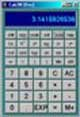# Ohms Law Quiz

• After studying this section, you should be able to:
• Calculate basic problems involving Ohm’s Law
• • Use appropriate units and sub-units.
• • Use a scientific calculator with engineering notation.

# Volts Amperes & Ohms### Fig. 3.2.1 The Ohm’s Law Triangleor (if you prefer) A ‘VIR’ Tree

Try a few calculations based on Ohms Law. For these you just need to use the three basic formulae described in Resistors & Circuits Module 3.1. Hopefully it´ll be a breeze. The important thing to remember is to use the correct version of the formula, and to get it the right way up (I = V/R is good but I = R/V is definitely NOT!). A simple visual aid to remembering the correct formula is the Ohms Law Triangle. Setting out V, I and R in this way is a reminder that V = IR (I multiplied by R), and I = V over (divide by) R and R = V over (divide by)I as shown in Fig 3.2.1.

Work out the answers using pencil and paper; if you don't write out the problem you WILL get mixed up half way and end up with the wrong answer. Of course the answer is not just a number, it will be a certain number of Ohms or Volts or Amperes, but wait, there is worse to come - those Ohms Volts or Amperes are very probably going to be kilohms or millivolts or microamperes, right? So you have to show that in your answer. No good just writing 56. Fifty six What? maybe 56Ω? Well if the real answer was 56KΩ you are still wrong, your answer is a thousand times too small!But don't worry, to get you off on the right track you should download our "Maths Tips" booklet, which shows you how to use your calculator with exponents and engineering notation to get the right answer every time.

Not got a scientific calculator? The "Maths Tips" booklet explains what you need (and what you don't need so you don't spend your money unnecessarily). If you don't want to buy a scientific calculator, you can always pick up a free one on the net. PC users can try Calc98 from www.calculator.org/download.html. Whichever calculator you choose, read the instructions to become familiar with the working methods you should use as these do vary from calculator to calculator.

OK so now you have read these instructions, you are ready to start. Here is a way to set out a typical problem on paper so you (with practice) don't get confused.

First write down what is known from the question, and what is not known:

V = ? (V is the unknown quantity.)

I = 500mA (500 x 10-3Amperes) 500E-3 or 500EXP-3 when entering it into your calculator, depending on which model you use.

R = 50Ω

So given I and R the correct formula to find V can be found from the Ohm´s law triangle:

V= I x R so substituting the figures given in place of I and R gives:

V = 500E-3 x 50 (for E press the E, the EE or the EXP key and for - press the change sign +/− or (-) key, NOT the minus (-) key. So the calculator display should read:

V = 500E-3 x 50 and pressing = gives the answer 25

The correct answer is therefore 25V

Note: If you are using Calc98 for your calculations you need to set the View>Option>Display menu to Engineering (under the "Decimal" choices).

It would be a good idea whilst you are in this menu to select 2 from the "Decimals" drop down box to set the number of digits displayed after the decimal place. This will round your answer down to two decimal places, which is sufficiently accurate for most uses and stops you getting silly answers such as 4.66666666667µA, which would be too accurate measure in a practical situation!

## Ohm´s Law Calculations Practice - Resistance, Voltage and Current.

### 1.

What will be the potential difference across a 50Ω resistor if a current of 500mA is flowing through it?

### 2.

What current will be needed to produce a voltage of 5V cross a 12kΩ resistor?

### 3.

What value of resistor will be needed to produce a current of 100mA when a voltage of 12V is applied across the resistor?

### 4.

What voltage will be developed across a 560Ω resistor if a current of 20mA is flowing through it?

### 5.

What current passing through 10kΩ resistor will produce a voltage of 8V cross it?

Top of Page.>